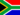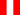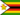# Historical cost and non-current asset valuation

• Study Reminders

We'll email you at these times to remind you to study

You can set up to 7 reminders per week

#### You're all set

We'll email you at these times to remind you to study

Monday

Tuesday

Wednesday

Thursday

Friday

Saturday

Sunday

• Study Notes
• Discuss This TopicSamuel F. 1 0 The advantage of using historical cost is that it is based on objective evidence rather than subjective opinion. However, the problem with some non-current assets such as property is that they usually increase in value over a number of years. In accounting - it is familiar understanding that different methods of arriving at a result or outcome exist. Asset valuation, which is critical in financial accounting, is not always uncomplicated. Asset values change yearly, due to depreciation and appreciation, and the nature of assets make asset valuation methods major. Four basic methods of valuation of assets in financial statement include: (I) Historical cost (II) Replacement cost (III) Net realizable value (IV) Economic value Historical cost is the most common valuation of assets in financial statements. This is because historical cost is provable and known. As its name suggests, historical cost recorded cost of an asset when the cost was incurred. In other words, the amount that an entity pays for an asset represents the historical cost. If, for case in point, you purchased an item for \$10,000.00, then it would be valued at its cost. Although financial accountants find "costs" a more objective index than asset values, asset valuations are important for fairly presenting the position of a business.Zachary B. 1 0 What do accounting issues usually deal with?Diamond T. 1 0 The advantage of using historical cost is that it is based on objective evidence rather than subjective opinion. However, the problem with some non-current assets such as property is that they usually increase in value over a number of years. In accounting - it is familiar understanding that different methods of arriving at a result or outcome exist. Asset valuation, which is critical in financial accounting, is not always uncomplicated. Asset values change yearly, due to depreciation and appreciation, and the nature of assets make asset valuation methods major. Four basic methods of valuation of assets in financial statement include: (I) Historical cost (II) Replacement cost (III) Net realizable value (IV) Economic value Historical cost is the most common valuation of assets in financial statements. This is because historical cost is provable and known. As its name suggests, historical cost recorded cost of an asset when the cost was incurred.Douglas R. 1 0 History and assets.Erick V. 0 0 debemos entender como costo histórico , el costo usual para un periodo productivo el mismo que esta sujeto en el futuro al dinamismo de la gestión y a políticas gubernamentales y también la depreciación y plusvalizacion ejemplo las propiedadesPride C. 1 0 The advantage of using historical cost is that it is based on objective evidence rather than subjective opinion. However, the problem with some non-current assets such as property is that they usually increase in value over a number of years. In accounting - it is familiar understanding that different methods of arriving at a result or outcome exist. Asset valuation, which is critical in financial accounting, is not always uncomplicated. Asset values change yearly, due to depreciation and appreciation, and the nature of assets make asset valuation methods major. Four basic methods of valuation of assets in financial statement include: (I) Historical cost (II) Replacement cost (III) Net realizable value (IV) Economic value Historical cost is the most common valuation of assets in financial statements. This is because historical cost is provable and known.
• Text Version